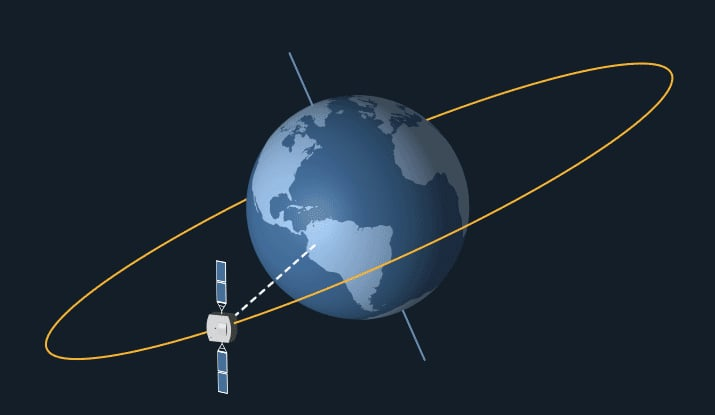PHY C8: Circular Orbits

We’ll be covering:

• Circular Orbits
• Components
• Kepler’s 3rd Law of Planetary Motion
• Types of Orbit

Let’s go!

Circular Orbits
We’ve already seen how circular motion works.
Let’s talk about circular orbits caused by gravitational force.

What are the components of circular orbits?

 Centre of Circular Motion Centre of mass of larger mass (e.g. planet) Radius, r Radius of planet + altitude of satellite r = R + h Period, T Time taken to complete one revolution. For example: the period of the Earth around the Sun = 365 days the period of the Moon around the Earth = 28 days You can use these values to calculate: ω = angular speed v = linear speed Centripetal Force, Fc Gravitational Force F = GMm/r2 Centripetal Acceleration, ac Gravitational Field Strength a = g = GM/r2

How are these all related?

 Fc = Fg RELATION 1: mv2/r = GMm/r2 Linear speed = circumference of orbit/period v = 2πr/T mv2/r = GMm/r2 v2 = GM/rSince g = GM/r2, RELATION 2: v2 = rg From relation 1: m(2πr/T)2/r = GMm/r2Rearranging: RELATION 3: T2/r3 = 4π2/GM From Relation 3: For different objects orbiting the same body (e.g. planets in a solar system), M is the same (mass of the Sun).Thus, it can be concluded that: T2 ∝ r3 “The square of the period is proportional to the cube of the orbital radius” This is Kepler’s 3rd Law of Planetary Motion.

Let’s talk about a few types of Earth orbit:Low Earth Orbit Satellites orbiting close to the vicinity of Earth. Their altitude is relatively small enough, such that r ≈ R.Thus, by calculation: v ≈ √(6.4 x 106 x 9.8) ≈ 8000 m/s T = 2πR/v ≈  5000 s Geostationary Orbit Satellites in equatorial orbits which the same period of rotation as the Earth. Thus, they are stationary in the sky to an observer on the ground.Credit: NASA T = TEarth = 24 hours This information can be used to calculate other aspects of motion: T2/r3 = 4π2/GM Plugging in T = 24 hrs, M = mass of the Earth, r = 4.23 x 107 h = r – R = 3.59 x 107 m v = 2πr/T Plugging in T = 24 hrs, v = 3070 ms-1

One thought on “PHY C8: Circular Orbits”

1.Irfan

the photo from Aerospaceweb has an error – centripetal acceleration should be towards the Earth, not tangential to the motion

Like